华东师范大学 985 双一流 211

East China Normal University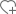关注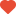已关注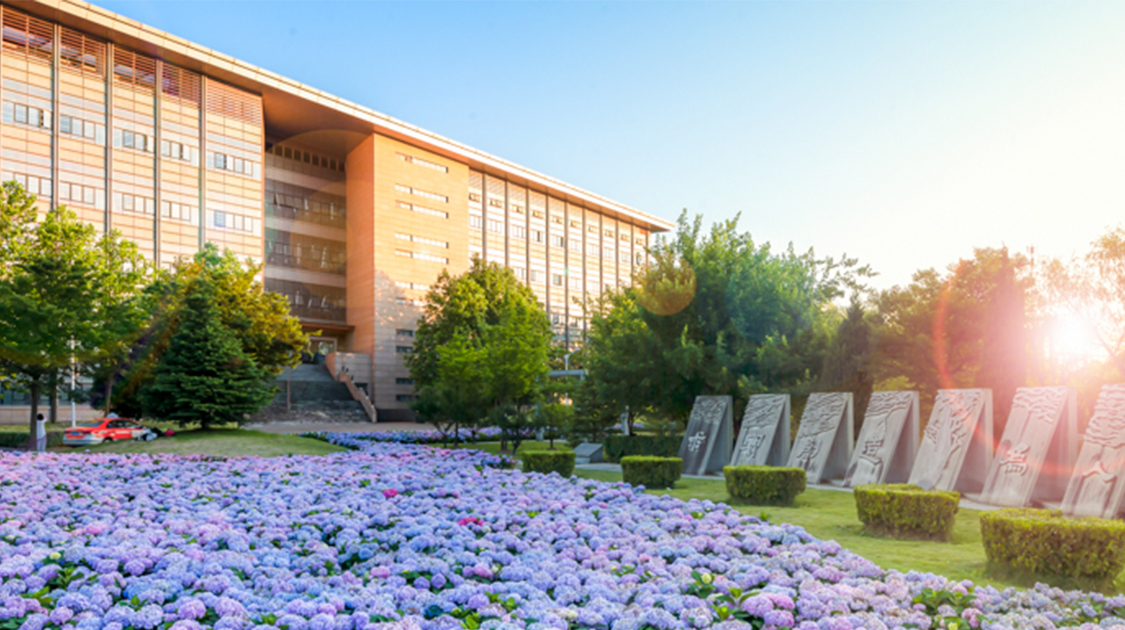•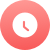1951

•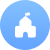公立

•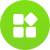师范

•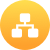教育部

•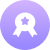本科

•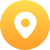上海-普陀区

•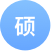227个

•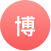153个

院校分数线

多方向招生特色专业

• 汉语言文学
• 历史学
• 心理学
• 软件工程
• 软件工程(嵌入式系统方向)
• 数学与应用数学
• 地理科学
• 对外汉语
• 化学
• 生物科学
• 哲学
• 学前教育
• 英语
• 体育教育
• 统计学院系/专业

• 地理科学（本）

• 生态学（本）
• 生态环境工程（本）
• 环境科学（本）
• 环境工程（本）

• 人文地理学（本）

• 学前教育（本）
• 艺术教育（本）
• 特殊教育（本）
• 特殊教育(心理)（本）
• 教育康复学（本）
• 公共事业管理（本）
• 教育技术学（本）
• 学前教育(公费师范)（本）

• 工商管理(中法创新实验班)（本）

• 金融学（本）
• 经济学（本）
• 国际经济与贸易（本）

• 工商管理（本）
• 会计学（本）
• 旅游管理（本）
• 会展经济与管理（本）
• 信息管理与信息系统（本）
• 房地产开发与管理（本）

• 人力资源管理（本）
• 行政管理（本）

• 统计学（本）
• 金融工程（本）
• 保险学（本）

• 汉语言文学（本）
• 历史学（本）
• 哲学（本）
• 政治学与行政学（本）
• 公共关系学（本）
• 思想政治教育（本）

• 思想政治教育（本）

• 法学（本）

• 社会学（本）
• 社会工作（本）

• 英语（本）
• 日语（本）
• 法语（本）
• 德语（本）
• 俄语（本）
• 英汉翻译（本）
• 西班牙语（本）

• 汉语国际教育（本）

• 心理学(师范、非师范基地班)（本）
• 应用心理学（本）

• 运动训练（本）
• 体育教育（本）
• 社会体育指导与管理（本）

• 广播电视编导（本）
• 播音与主持艺术（本）
• 新闻学（本）
• 广告学（本）
• 编辑出版学（本）

• 音乐学（本）
• 绘画（本）

• 美术学（本）
• 雕塑（本）

• 视觉传达（本）
• 产品设计（本）
• 环境艺术（本）
• 公共艺术（本）
• 时尚设计（本）

• 数学与应用数学（本）
• 信息与计算科学（本）

• 物理学（本）
• 电子科学与技术（本）

• 化学(师范)（本）
• 应用化学（本）

• 生物科学（本）
• 生命医学（本）

• 微电子科学与工程（本）
• 电子信息科学与技术（本）
• 通信工程（本）

• 计算机科学与技术（本）
• 软件工程（本）

• 数据科学与工程（本）

大学排名知名校友

•李彦宏

•俞洪敏

•撒贝宁

•马云400-181-5008

(免长话费 工作日 09:00-17:30)